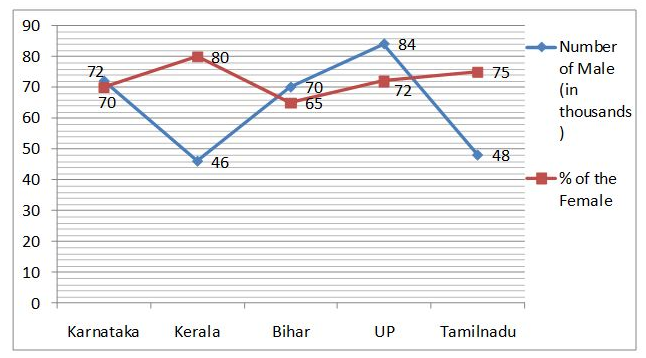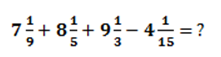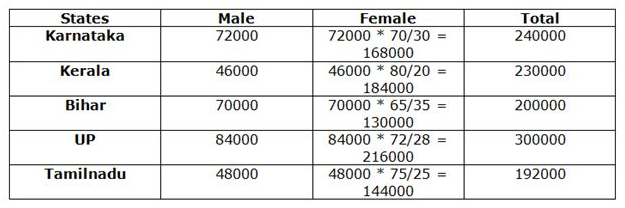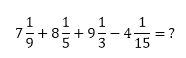# RRB Clerk Mains Quantitative Aptitude Questions 2021 (Day-02)

Dear Aspirants, Our IBPS Guide team is providing new series of Quantitative Aptitude Questions for IBPS RRB Clerk Mains 2019 so the aspirants can practice it on a daily basis. These questions are framed by our skilled experts after understanding your needs thoroughly. Aspirants can practice these new series questions daily to familiarize with the exact exam pattern and make your preparation effective.

Start Quiz

Line graph

Directions (01-05): Study the following information carefully and answer the questions given below.

The given line graph shows the number of male population (in thousands) and the percentage of the female population in five different states.1) Ratio of the number of illiterate population to literate population in Karnataka is 3:5. If the ratio of the number of male literate to illiterate population in Karnataka is 5:3, then what is the ratio of the number of literate to illiterate female population in Karnataka?

A.4:3

B.5:3

C.3:2

D.3:1

E.6:5

2) Number of male population in Haryana is 50% more than the number of male population in Tamilnadu and the number of female population in Haryana is 25% more than the number of female population in Kerala. What is the total number of population in Haryana?

A.296000

B.300000

C.302000

D.306000

E.310000

3) What is the average number of female population in all the states together?

A.168500

B.168700

C.168400

D.168200

E.168900

4) The average number of population in Kerala and UP is approximately what percent of the average number of population in Bihar and Tamilnadu?

A.135%

B.138%

C.142%

D.145%

E.148%

5) What is the difference between the average number of male population in Karnataka, Tamilnadu and UP together and the average number of female population Tamilnadu and UP?

A.114000

B.115000

C.117000

D.112000

E.116000

Simplification

Directions (06-10): What value should come in the place of question mark (?) in the following questions?

6) ? = (14 * (13²+131))/40 + 60% of 400

A.325

B.345

C.260

D.275

E.None of these

7) (18 * 24 – 13²) + 40% of (18 * 25) =?

A.392

B.413

C.420

D.440

E.443

8) 234.5453 + 34234.5342 + 3324.234 + 4532.2314 = ?

A.42335.5449

B.52325.5449

C.42325.5449

D.43325.5449

E.none of these

9) 35% of 46% of 50% of 24% of 11150 = ?

A.241.815

B.215.418

C.214.518

D.124.815

E.none of these

10)A.10(26/45)

B.22(26/45)

C.20(24/45)

D.20(26/45)

E.none of these

Directions (1-5) :Number of literate population in Karnataka = 240000 * 5/8 = 150000

Number of illiterate population in Karnataka = 3/8 * 240000 = 90000

Number of male literate population in Karnataka = 5/8 * 72000 = 45000

Number of male illiterate population in Karnataka = 3/8 * 72000 = 27000

Required ratio = (150000 – 45000):(90000 – 27000)

= 105000:63000

= 5:3

Required total = 150/100 * 48000 + 125/100 * 184000

= 72000 + 230000

= 302000

Required average = (168000 + 184000 + 130000 + 216000 + 144000)/5

= 842000/5

= 168400

Required percentage = ((230000 + 300000)/2)/((200000 + 192000)/2) * 100

= ((530000/2)/(392000/2)) * 100

= 265000/196000 * 100

= 135%

Required difference = (216000 + 144000)/2 – (72000 + 48000 + 84000)/3

= (360000/2) – (204000/3)

= 180000 – 68000

= 112000

X = (14 * (13²+131))/40 + 60% of 400

X = (14 * 300)/40 + 60/100 * 400

= 105 + 240

=345

X = (18 * 24 – 13²) + 40/100 * (18 * 25)

= (432 – 169) + 2/5 *(450)

= 263 + 180

= 443

234.5453 + 34234.5342 + 3324.234 + 4532.2314 = 42325.5449

35/100 x 46/100 x 50/100 x 24/100 x 11150 = 215.418= (7 + 8 + 9 – 4) + (1/9 + 1/5 + 1/3 – 1/15)

= 20 + (5 + 9 + 15 – 3)/45

= 20 + 26/45

= 20(26/45)

5 1 vote
Rating